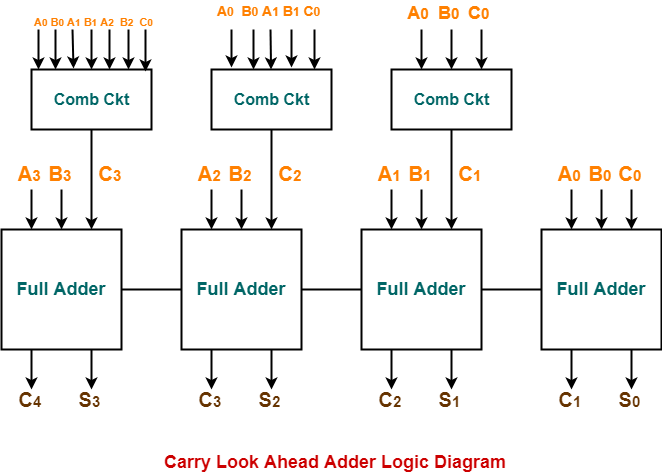2,929 views

In which of the following adder circuits, the carry look ripple delay is eliminated?

### 1 comment

carry look ripple delay is eliminated in Carry-look-ahead adder as carry is already provided before the operation starts .

carry is calculated as follows:-Substituting C1 into C2, then C2 into C3, then C3 into ​​​​​​​C4 yields the following expanded equations:Here G (carry generator) is equal toP(propagate) as followsall the carry are calculated using the above formula and provided to input as mentioned in a diagram. Thus ripple delay is eliminated.Option D; as in CLA the carry is generated in parallel. Hence no concept of ripple carry.

Please refer to the carry equationWhere $Gi$ is the generator Function and $Pi$ is the propagation function.
$Gi$ = $AiBi$ (Ai,Bi are Bit pairs of the two n bit numbers)
$Pi$ = $Ai+Bi$ or $Ai ⊕ Bi$
Follow CLA for further clarification.
by
option (d) is correct.

1 vote
1
752 views